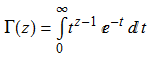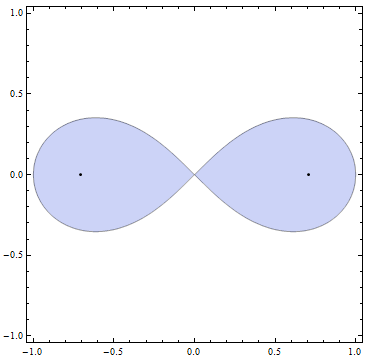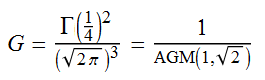# Gamma(1/4)

The Gamma function, Γ, is an extension of the factorial function to the complex plane. Specifically, Γ(n) = (n−1)! for all positive integers n. It is defined, more generally, by analytic continuation of the following integral, which converges for all complex numbers with positive real part:The factorial identity can be verified using integration by parts. Specifically, we get the recurrence relation Γ(z+1) = z Γ(z). Plotting the absolute value of the function in the complex plane yields the following surface, with poles situated at zero and the negative integers:At certain non-integer arguments, we get new irrational constants. For example, Γ(1/2) is the square-root of π. Γ(1/4) is even more interesting, and has been shown (by Yuri Nesterenko, in 1996) to be algebraically independent of both π and e^π; in other words, there is no non-trivial polynomial in π, e^π and Γ(1/4) with integer coefficients that evaluates to zero.Also, we have$\Gamma(\frac{1}{4})^2 = S \sqrt{2 \pi}$, where S is the arc length of the lemniscate (shown above) given by the equation$(x^2 + y^2)^2 = x^2 - y^2$ (and, of course,$\sqrt{2 \pi}$ is our favourite transcendental constant). The area bounded by the curve is precisely 1, otherwise known as Legendre’s constant.

Related to this is Gauss’s constant:AGM is the arithmetic-geometric mean, which is obtained by repeatedly iterating the map (x, y) → (√(xy), ½(x + y)) and taking the limit. There is an algorithm (the Brent-Salamin method) for calculating pi based on the arithmetic-geometric mean. The AGM converges extremely quickly, producing exponentially many digits of precision after n iterations. Nevertheless, the slower Chudnovsky algorithm is preferred for computing pi, due to the inefficiency of computing square roots.

This entry was posted in Uncategorized. Bookmark the permalink.

### 0 Responses to Gamma(1/4)

1.Kasuha says:

I always thought that complexity of multiplication, division, and square root is about the same… but then I realized there are ways of calculating multiplication which go below O(N^2) where N corresponds to precision.